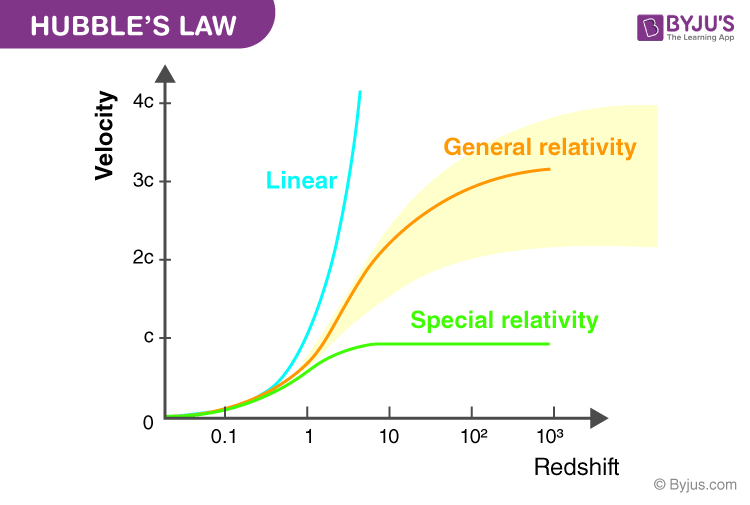Checkout JEE MAINS 2022 Question Paper Analysis : Checkout JEE MAINS 2022 Question Paper Analysis :

# Hubble’s Law

## What is Hubble’s Law?

Hubble’s law statement is given as

The velocity of the galaxy which is also known as the redshift is directly proportional to its distance.

Hubble’s law is also known as Hubble-Lemaitre law, studied in physical cosmology. Only with the help of Hubble’s law the universe can expand and fulfill the cosmological principle.

## Hubble’s Law Formula

Hubble’s law formula is given in the following table:

 $$\begin{array}{l}v = H_{0}d\end{array}$$

Where,

• v is the velocity of the galaxy in km/s
• H0 is the Hubble constant in km/s/Mpc
• d is the distance of the galaxy in Mpc

## Limitations of Hubble’s Law

By determining the shift in observed light into redshift in the spectrum, one can determine the distance of the galaxy from us using Hubble’s equation after measuring the recession velocity. Following are the limitations of Hubble’s law which makes the measurement challenges:

• Because of the intrinsic motion of galaxies, observed velocity gets influenced.
• Galaxy orbiting due to gravitational movements.

### What is Hubble Constant?

Hubble constant is defined as the unit of measurement, which is used for describing the expansion of the universe. Hubble’s constant H is 160 km/s per million-light-years.

### What is Redshift?

Redshift is the phenomenon in which an object’s wavelength increases due to electromagnetic radiation. Blueshift is opposite to redshift where the energy increases due to shorter wavelengths which are also known as negative redshift. The main reasons for redshift are as follows:

• Doppler effect ie; the movement of objects either closer or apart from each other in space.
• Strong gravitational force leads to gravitational redshift.
• Cosmological redshift is an expansion of space such that objects are separated without the change in their position.

#### Interpretation of Redshift And DistanceAbove is the linear relationship between the redshift and distance and the mathematical expression for Hubble’s law as follows:

v = HοD

Where,

• v is the recessional velocity in km/s
• Hο is the Hubble’s constant
• D is the proper distance between the galaxy and the observer

Redshift formula

### Redshift Formula

The redshift formula is given in the below table:

 $$\begin{array}{l}z = \frac{\Delta \lambda }{\lambda }\end{array}$$

Where,

• z is the redshift
• Δλ is the shift in wavelength in the spectra
• λ is the wavelength

### Example of Hubble’s Law

Q1. For some cluster, velocity is measured as v = 103 km/s. What is the distance? Assume Hο = 60km/s/Mpc
Ans: We know,
v = 103 km/s
H0 = 60km/s/Mpc
The formula used is:
d = v/Hο
=(103 km/s)/(60km/s/Mpc)
=16.7 Mpc

## Units Derived From Hubble Constant

There are three units that are derived from Hubble constant, and they are:

• Hubble Time

The Hubble time is defined as the age taken by the universe to expanded if the expansion was linear and in different real age. This is dependent on the dimensionless factor which is dependent on the mass and energy of the content in the universe.

Hubble time is given as follows:

tH = 1/Hο = 1/67.8 (km/s)/Mpc = 4.55.1017s = 14.4 billion years

• Hubble Length

The Hubble length is defined as the product of the speed of light and the Hubble time. It is also known as the Hubble distance. The product obtained is equivalent to 14.4 billion light-years.

$$\begin{array}{l}cH_{0}^{-1}\end{array}$$
is the Hubble length.

• Hubble Volume

The Hubble volume is defined as the volume of the observable universe. It is also defined as the volume of sphere whose radius is

$$\begin{array}{l}cH_{0}^{-1}\end{array}$$
or a square with side
$$\begin{array}{l}cH_{0}^{-1}\end{array}$$
.

Related Physics Articles

To read such interesting physics articles, subscribe BYJU’S app today!

## Frequently Asked Questions – FAQs

### What is Hubble’s law used for?

Hubble’s law is used for understanding the motion of the astronomical objects due to the expansion of the universe. This was first used for studying the expansion of the universe and is used as the evidence for the big bang model.

### In what situations does Hubble’s law not apply?

Hubble’s law is not applicable for understanding the motion of stars in the Milky Way galaxy or other heavenly objects in the Solar system. It is applicable to distant galaxies.

### Why is Hubble’s law so important?

Hubble’s law is considered to be so important because of the value of Hubble constant, H which is used for determining the exact age of the universe. It also helps in understanding the Dark matter and Dark energy.

### What is the value of Hubble’s constant?

The value of Hubble’s constant was determined by Planck and found it to be equal to 46,200 mph per million light-years.

### Why is Hubble’s constant uncertain?

Hubble’s constant is said to be uncertain as to the constant changes with time. However, the expansion of the universe is constant throughout the space and at every location. This is the reason why it is called Hubble’s constant.

Test Your Knowledge On Hubbles Law!